# Dodecagon

Regular dodecagon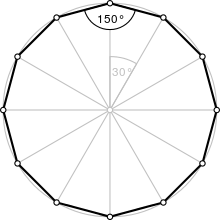A regular dodecagon
Type Regular polygon
Edges and vertices 12
Schläfli symbol {12}, t{6}, tt{3}
Coxeter diagram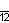Symmetry group Dihedral (D12), order 2×12
Internal angle (degrees) 150°
Dual polygon Self
Properties Convex, cyclic, equilateral, isogonal, isotoxal

In geometry, a dodecagon or 12-gon is any twelve-sided polygon.

## Regular dodecagon

A regular dodecagon is a closed figure with sides of the same length and internal angles of the same size. It has twelve lines of reflective symmetry and rotational symmetry of order 12. A regular dodecagon is represented by the Schläfli symbol {12} and can be constructed as a truncated hexagon, t{6}, or a twice-truncated triangle, tt{3}. The internal angle at each vertex of a regular dodecagon is 150°.

### Area

The area of a regular dodecagon of side length a is given by:And in terms of the apothem r (see also inscribed figure), the area is:In terms of the circumradius R, the area is:The span of the dodecagon is the distance between two parallel sides and is equal to twice the apothem. A simple formula for area (given side length and span) is:This can be verified with the trigonometric relationship:### Perimeter

The perimeter of a regular dodecagon in terms of apothem is:The perimeter in terms of circumradius is:This coefficient is double the coefficient found in the apothem equation for area.

## Dodecagon construction

As 12 = 22 × 3, regular dodecagon is constructible using compass and straightedge: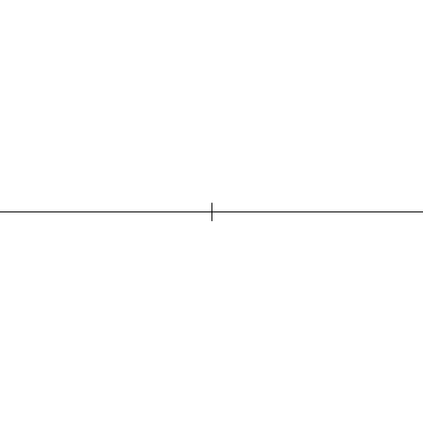Construction of a regular dodecagon at a given circumcircle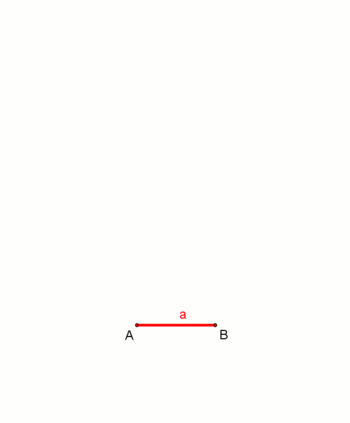Construction of a regular dodecagon
at a given side length, animation. (The construction is very similar to that of octagon at a given side length.)

## Dissection

Coxeter states that every parallel-sided 2m-gon can be divided into m(m-1)/2 rhombs. For the dodecagon, m=6, and it can be divided into 15 rhombs, with one example shown below. This decomposition is based on a Petrie polygon projection of a 6-cube, with 15 of 240 faces.With hexagons, squares, and triangles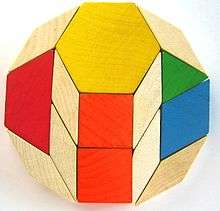pattern blocks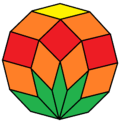With 15 rhombs from 6-cube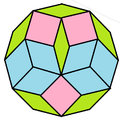With 15 rhombs

One of the ways the mathematical manipulative pattern blocks are used is in creating a number of different dodecagons.

## Symmetry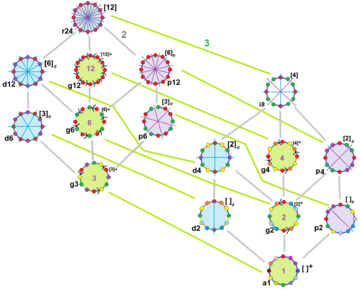The symmetries of a regular dodecagon as shown with colors on edges and vertices. John Conway labels these lower symmetries with a letter and order of the symmetry follows the letter. He gives d (diagonal) with mirror lines through vertices, p with mirror lines through edges (perpendicular), i with mirror lines through both vertices and edges, and g for rotational symmetry. a1 labels no symmetry. These lower symmetries allows degrees of freedoms in defining irregular dodecagons.

The regular dodecagon has Dih12 symmetry, order 24. There are 15 distinct subgroup dihedral and cyclic symmetries. Each subgroup symmetry allows one or more degrees of freedom for irregular forms. Only the g12 subgroup has no degrees of freedom but can seen as directed edges.

## Occurrence

### Tiling

A regular dodecagon can fill a plane vertex with other regular polygons in 4 ways:

3.12.12 4.6.12 3.3.4.12 3.4.3.12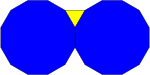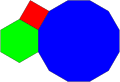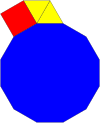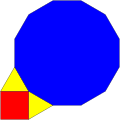Here are 3 example periodic plane tilings that use regular dodecagons, defined by their vertex configuration:

1-uniform 2-uniform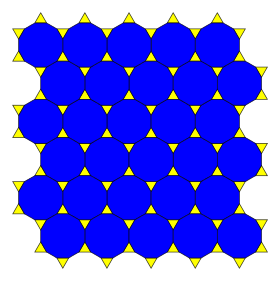3.12.12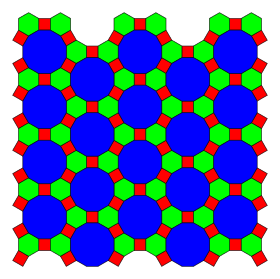4.6.12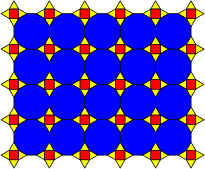3.12.12; 3.4.3.12

## Skew dodecagon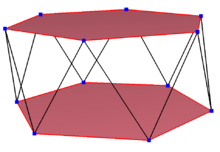A regular skew dodecagon seen as zig-zagging edges of a hexagonal antiprism.

A skew dodecagon is a skew polygon with 12 vertices and edges but not existing on the same plane. The interior of such an dodecagon is not generally defined. A skew zig-zag dodecagon has vertices alternating between two parallel planes.

A regular skew dodecagon is vertex-transitive with equal edge lengths. In 3-dimensions it will be a zig-zag skew dodecagon and can be seen in the vertices and side edges of a hexagonal antiprism with the same D5d, [2+,10] symmetry, order 20. The dodecagrammic antiprism, s{2,24/5} and dodecagrammic crossed-antiprism, s{2,24/7} also have regular skew dodecagons.

### Petrie polygons

The regular dodecagon is the Petrie polygon for many higher-dimensional polytopes, seen as orthogonal projections in Coxeter planes. Examples in 4 dimensionare the 24-cell, snub 24-cell, 6-6 duoprism, 6-6 duopyramid. In 6 dimensions 6-cube, 6-orthoplex, 221, 122. It is also the Petrie polygon for the grand 120-cell and great stellated 120-cell.

## Related figures

A dodecagram is a 12-sided star polygon, represented by symbol {12/n}. There is one regular star polygon: {12/5}, using the same vertices, but connecting every fifth point. There are also three compounds: {12/2} is reduced to 2{6} as two hexagons, and {12/3} is reduced to 3{4} as three squares, {12/4} is reduced to 4{3} as four triangles, and {12/6} is reduced to 6{2} as six degenerate digons.

Deeper truncations of the regular dodecagon and dodecagrams can produce isogonal (vertex-transitive) intermediate star polygon forms with equal spaced vertices and two edge lengths. A truncated hexagon is a dodecagon, t{6}={12}. A quasitruncated hexagon, inverted as {6/5}, is a dodecagram: t{6/5}={12/5}.

## Examples in use

In block capitals, the letters E, H and X (and I in a slab serif font) have dodecagonal outlines. A cross is a dodecagon.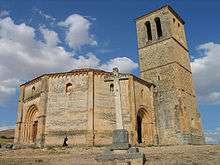The Vera Cruz church in Segovia

The regular dodecagon features prominently in many buildings. The Torre del Oro is a dodecagonal military watchtower in Seville, southern Spain, built by the Almohad dynasty. The early thirteenth century Vera Cruz church in Segovia, Spain is dodecagonal. Another example is the Porta di Venere (Venus' Gate), in Spello, Italy, built in the 1st century BC has two dodecagonal towers, called "Propertius' Towers".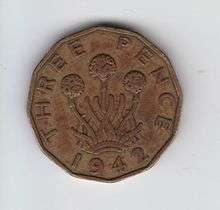A 1942 British threepence, reverse

Regular dodecagonal coins include: